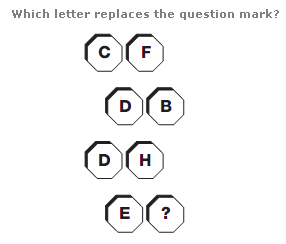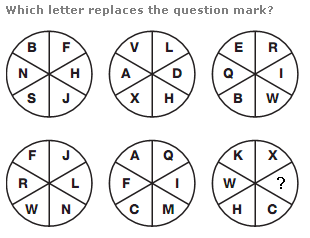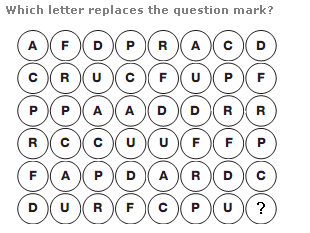# Puzzles - Missing letters puzzles

### Exercise :: Missing letters puzzlesAnswer : D Explanation : Converting each letter to its numerical value and reading each line as a 2 digit number, the numbers follow the sequence of multiples of 6, from 36 to 54.Answer : O Explanation : Starting with the left hand circle in the top row, add 4 to the numerical value of each letter and write the letter with this value in the corresponding segment of the lower left circle. Continue this pattern, adding 5 to the letter values in the central circle, and 6 in the right hand circle.Answer : A Explanation : Start at the top left of the diagram, and work down the left hand column, then along one space to the right and up the next column, in a snakes and ladders pattern, write the following repeated sequence of letters: A C P R F D U